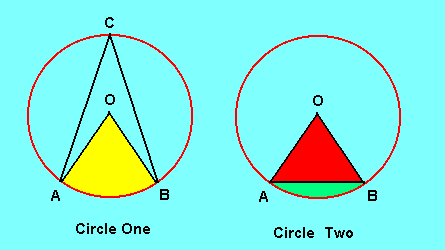# Circle Information Calculator

This calculator requires the use of Javascript enabled and capable browsers. This calculator is designed to give information about a given circle from the entered values of the central angle and the radius. From the two circles, the following is given information:

Lines AO and OB are called radii. One of them is a radius. They are lines from the outer edge of the circle to the center.
Lines AC, BC and AB are called chords. They are lines inside the circle from the outside surface to any other outside surface.
The angle ACB is an inscribed angle.
The angle AOB is a central angle.
The curved line AB is called an arc.
The length of an arc equals (Central Angle / 180°)• p · r
The yellow area that looks like a slice of pie is a sector.
The area of a sector equals (Angle AOB / 360°)• p · r˛
The green area in the second circle is a segment.
The area of a segment equals Area of the Sector Minus the Area of Triangle AOB.Unit Number Required Data Entry Central Angle° Radius Calculated Results Arc Length Chord AB Sector Area Segment Area Triangle Area Triangle Height Total Circle Area
Version 1.3.6

 Leave us a question or comment on FacebookSearch or Browse Our Site